﻿ Series Primes in Binary

### Series Primes in Binary

P.M. MazurkinOPEN ACCESSPEER-REVIEWED

## Series Primes in Binary

Doctor of Engineering Science, Academician of RANS, member of EANS, Volga Region State Technological University, Russia

### Abstract

To prove the famous Riemann hypothesis, that the real part of the root is always exactly equal to 1/2, a series of 500 and the other prime numbers has been converted from decimal to binary number system. At the same time was a clear non-trivial zeros. Any prime number can be represented as quantized into binary digital signal. Quantization step to not dilute a number of prime numbers is 1. Number of levels (binary digits) depends on the power of the quantized number of primes. As a result, we get two types of zeros - the trivial and nontrivial. Capacity of a finite number of primes must be taken based on the completeness of block incidence matrix. Average statistical indicator is a binary number, and influencing variable - itself a prime number. The binary representation allows to visualize and geometric patterns in the full range of prime numbers.

### At a glance: Figures

1
Prev Next

• Mazurkin, P.M.. "Series Primes in Binary." American Journal of Applied Mathematics and Statistics 2.2 (2014): 60-65.
• Mazurkin, P. (2014). Series Primes in Binary. American Journal of Applied Mathematics and Statistics, 2(2), 60-65.
• Mazurkin, P.M.. "Series Primes in Binary." American Journal of Applied Mathematics and Statistics 2, no. 2 (2014): 60-65.

 Import into BibTeX Import into EndNote Import into RefMan Import into RefWorks

### 1. Introduction

The decimal number system in areal density inferior to many other systems of notation, but in convenience and in the force of habit in the frequency of use of man at the time of 02.07.2011 superior to other number system (from the Internet).

The binary number system for a number of primes should be the effective positioning system. It has only two whole numbers: 0 and 1 .

### 2. Quantization of a Simple Number

Any prime number can be represented as the quantum of the binary system of the sampled signal. In the quantization of the entire range of the signal is divided into levels, the amount of which shall be represented in the numbers of a given digit capacity . The distance between these levels is called the quantization step, and it is equal to 1 is not for the diluted number of primes. The number of levels (bits of the binary notation) depends on the power of the quantized number of primes.

500 prime numbers. We take a number of primes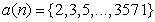at. Table 1 shows the fragments of quantization on the boundary crossings (frames) between the discharges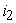of the binary system. Given on the left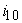of the decimal system.

Has long been known that the numbers that grow naturally, for example, such as powers of two, would, of course, absurd to look for an instance, surpassing all known. For simple numbers is making enormous efforts to do just that. Primes were factorization, i.e. expansion in numbers and multipliers with large powers of two. It is this passion and are not allowed mathematicians to use the binary system for the analysis no multipliers of the prime number, and quantized in binary terms.

### 3. Prime Properties

The hierarchy we consider several basic properties.

1. Any simple contains the number of bits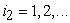of the binary system and components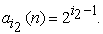(1)

2. Any prime number is the sum of the components of the given matrix incidence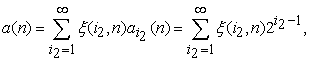(2)

where- is the incidence matrix, and always. For an infinite-dimensional of a number of primes have levels of quantization or area of digits in the binary system.

An example of calculations by formula (2) is given in Table 2.

As a result, we get two types of zeros - the trivial and nontrivial. The first are located, as seen from the two tables on the left to the vertical 1 in each block. A nontrivial zeros are located within a two-column with 1, where the left column 1 is shifted by blocks with the increase in prime number. In Table 1 the trivial zeros are shown empty cells.

3. The number of non-trivial zeros tends to infinity, because a number of quantization levels also tend to infinity in the conditions of,and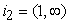.

#### Table 2. A number of prime numbers (fragment) in decimal notation

4. When the first digitof the binary number (Table 1) for the conditionsand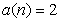is the incidence of, and for some non-critical primesincidence is equal to, where throughout theand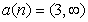. Critical primes require a separate study.

5. For non-critical primesandwill be adequate findings obtained on a finite number of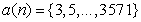at capacity.

### 4. Mathematical Landscape

In a remarkable series of films «De Code» (19.07; 26.07 and 08.02.2011) leading Mark Dyusotoy shows a graphical picture three-dimensional "mathematical landscape" Riemann zeta function. All pay attention to the non-trivial zeros on the critical line. They are already counted several trillion.

But we are attracted to this in the landscape of another - steep slopes rising at the approach. Alignment of the binary system are infinitely high "mountain" makes projections of equal height, equal to one. Figure 1 shows a three-dimensional graph, for clarity, built only in the part of one block of 20 primes.

In Figure 1 appears a certain ceiling from units, except "floor" from the nontrivial zeros. Between them there is an unknown relationship. Then a super Riemann surface, due to the presentation of complex numbers, converted to a double-layer "cake".

Figure 1. Mathematical landscape fragment of of the 20 prime numbers from 1031 to 1163

Have to consider these two layers along (in the order of simple numbers) and across (per grade). For the analysis we introduce a parameter - the binary number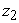that takes real values.

### 5. Binary Number along the Row

For the analysis of the data in Table 1 were taken only whole blocks of the incidence matrix, i.e. without trivial zeros. For the columnwill delete the first row and then we get. This is the "mountain", from which in the transverse direction there will be non-trivial zeros. The fact that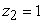the condition is unchanged for all infinite-length, let us consider in another article.

For(except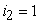) have average values (Table 3).

### 6. Effect of Discharge i2

After the identification of stable laws for 500 lines (without) was obtained (Figure 2) model(3)

In a four-distribution model, of the distribution of the average value of the binary number of the first component is the law of exponential death (of the slope of the landscape), and the second - the law of exponential growth, starting from the third digit binary number system. Then, additional oscillatory disturbances produce two waves of adaptation.

The first of these is the increasing amplitude, and shows that if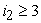half the amplitude of the binary number is increasing by the law of exponential growth.

The second wave through a dischargewill be close to zero. The picture changes completely with further increase in number of primes.

The maximum relative error of formula (3) at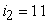is 1002.47674e-005 / 0.60366 = 0,0041 %. At the same time schedule is very similar to the Riemann zeta-function.

### 7. Blocks of Prime Numbers

Computational experiments showed that the power series should be taken based on the completeness of the block incidence matrix. For example, take the block number 11 with a fragment that has parameters:,,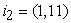. Comparison showed a significant power series of prime numbers, whose serial number has only secondary importance. The indicator is average statistical (but not the arithmetic mean) binary number, and the explanatory variable - itself a prime number.

Calculations based on block number 11 (Figure 3) are given in Table 4 and were performed according to the formula(4)

If we ignore the first and last bits binary system, the closest to a rational number 1/2 on the real values of the formula (4) is the discharge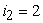.

As can be seen from the graphs in Figure 3, balances are close to zero only when the two digits 2 and 3. In other cases, they are all over the interval (-0.5, 0.5).

#### Table 4. Effect of the prime on the binary number of digits of the binary system

Figure 3. Graphs of statistical models (4) of the binary number: S - dispersion; r- correlation coefficient

### 8. Critical Zeros or Ones?

Equation (4) as we approach the second category is gradually reduced to the expression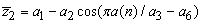, obtaining a constant frequency oscillations with half-periods of 2 or 4. Become critical as zero and one. Critical become as zeros so and units.

### 9. The Real Part 1/2.

From the Internet we know: "Here is the famous Riemann hypothesis, that the real part of the root is always exactly equal to 1/2, has not yet been proved, though it would have to prove the theory of prime numbers is extremely important."

Equation (4) proves that not only the real part of the root is 1/2, but there are other interesting results. For example, in the formulas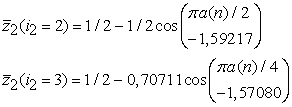(5)

expression in front of the cosine function on critical line is exactly equal to 1/2. Options 1,59217 and 1,57080 showing the shift of a wave with constant amplitude, very close to the irrational number, and the number 0,70711 is close to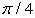. The emergence of a number of space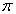transforms equation (4) in a model of spatial signal. It is characterized by a symmetric wavelet with constant amplitude of1/2 and variable frequency (in the formula - half-period).

Riemann zeta-function has zeros at the negative, even, multiples of 2. But the data in Table 3 show that the frequency of occurrence of the nontrivial zeros is. Then the Riemann obtained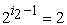only whenthat is exactly on the critical line.

Note also that the zeta-function in complex variables accepted function of sine, but cosine better for the real numbers, since it allows to ignore the signs in terms of a trigonometric function. Cosine works in both quadrants on a number of natural numbers.Therefore, it will be successful in a number of prime numbers.

### 10. The Algorithm of Predicting of a Simple Number

While we do not believe in the possibility of predicting the next term in the series of prime numbers. But, after their conversion to binary form, are clear boundaries between the blocks. Rules for translating decimal numbers to binary  is quite sufficient to explain the "jumps" in a series of prime numbers.

### 11. Asymptotic Frames

Capacity of a number of prime numbers is quite possible to manage. To do this, from Table 2 we write down the bold values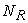(Table 4).

### 12. Conclusions

To prove the famous Riemann hypothesis, that the real part of the root is always exactly equal to 1/2, the transformation was a series of prime numbers from decimal to binary number system. At the same time become visible and non-trivial zeros.

### References

  Gashkov S.B. Number systems and their applications. M. MCCME, 2004. 52.In article  Signal. URL: http://ru.wikipedia.org/wiki/%D0%A1%D0%B8%D0%B3%D0%BD%D0%B0%D0%BB.In article